|

# 燕赵家博会2.22-24即将开幕,家居装修一站到位

一年之计在于春，又一轮装修旺季即将来袭，如何找到更具性价比的装修方案？如何一站式购物超所值的装修建材？跑百家不如逛一家！2月22日-24日，第十三届燕赵家博会将在石家庄国际会展中心（正定）盛大开幕，展出范围涵盖综合建材、装修公司、陶瓷卫浴、定制家具、橱柜厨电、家具家电等，覆盖家居装修全产业链，为家居建材经销商提供高效的营销平台，为广大业主提供放心、高效、钜惠的一站式家居建材采购平台。权威家装平台，品质装修首选

燕赵家博会，被石家庄市政府列为重点扶持展会，在社会各界的大力支持之下，依靠稳定的团队，强大的执行力和经验丰富的媒体资源整合应用能力，连续多年领跑河北省家居建材会展行业，是河北省规模最大、受众最广的家装建材类品牌展会、权威展会、放心展会。目前已成功举办了十二届，伴随多年在家居建材行业的持续耕耘，，已经累计服务超过80000个装修家庭，成交超过100000单，是石家庄广大业主品质装修采购首选平台。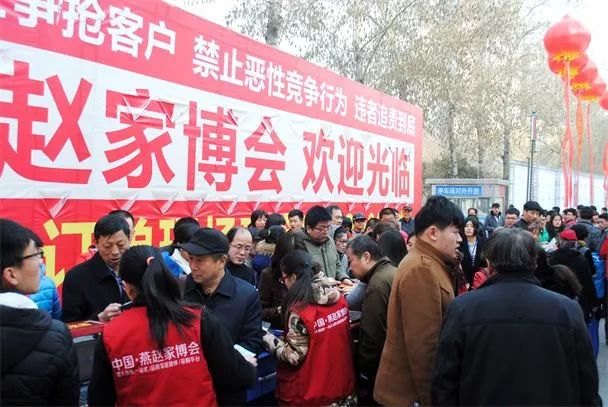全品类一线大牌云集，家居装修一站购齐

在参展品牌的选择上，此次家博会的参展商都经过了组委会的严格审核、层层筛选，凡参展的家装企业均是有资质且有实力、诚信度高、口碑好的优秀品牌商家；精挑细选的200余个“牌子响、专业性强、认可度高”的参展品牌，覆盖家居装修产业链全品类一线品牌，必将打造一场无以伦比的家居建材购物盛宴，满足广大业主一站式装修采购的需求。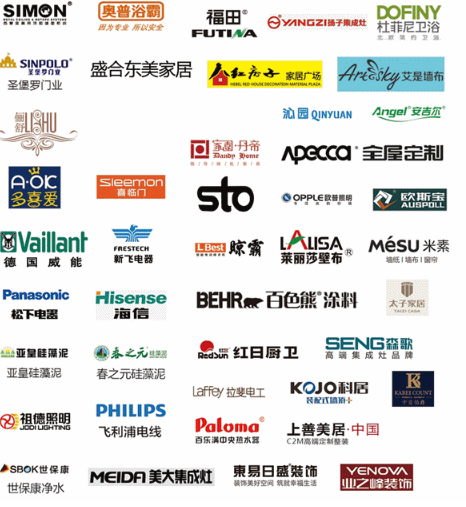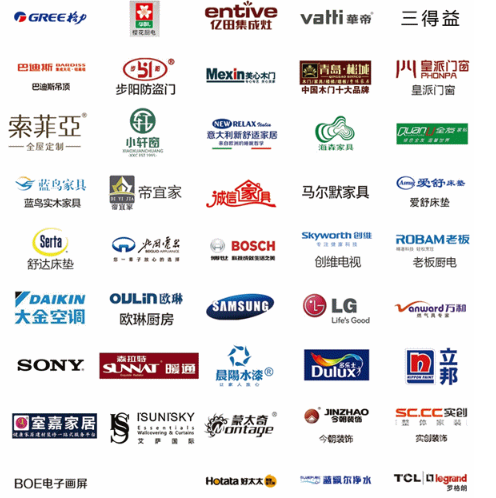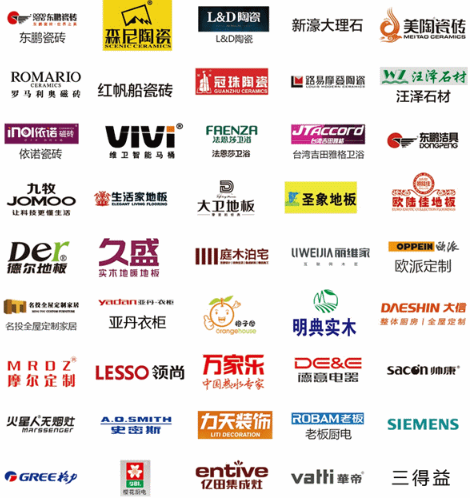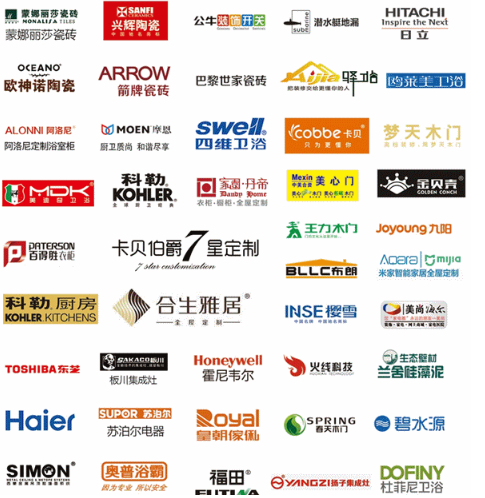值得一提的是，北国电器作为石家庄本土极具影响力的电器连锁卖场，将鼎力助阵燕赵家博会，携海尔、西门子、美的、方太等12家一线家电品牌重磅亮相，带来众多新款和热销产品，满足广大业主朋友不同层次的需求。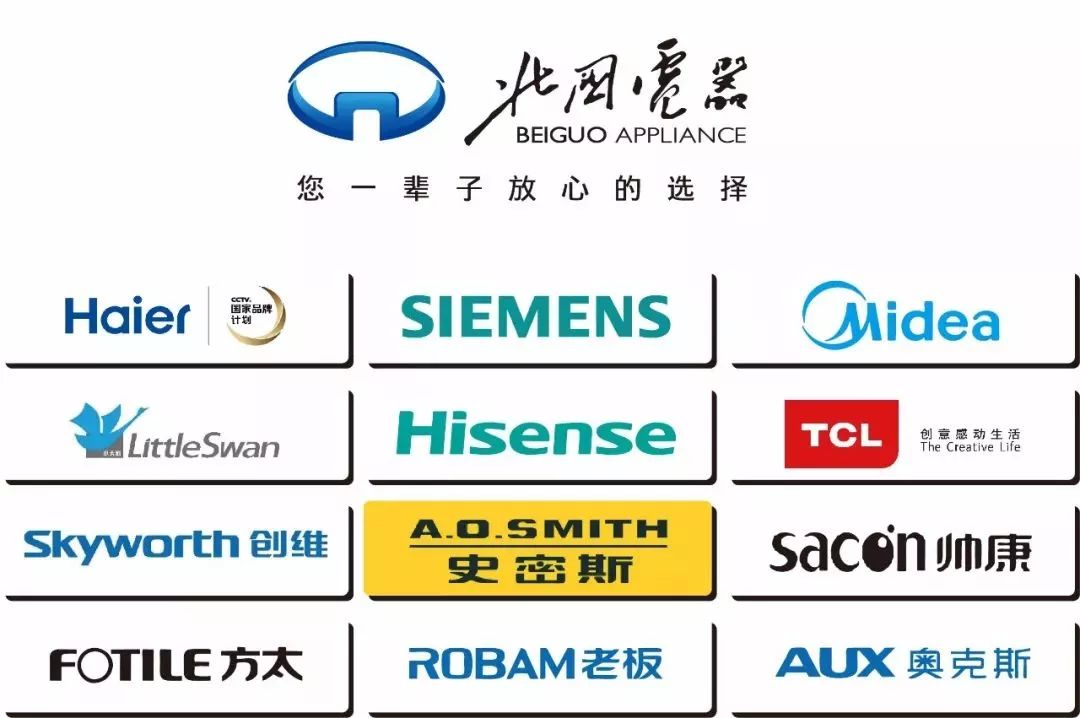疯狂让利大放价，品牌建材低价购

本届家博会参展商家大多是地区总代理，减少了中间环节确保底价，并且很多商家为了争创新春开门红，争取到厂家支持，打出“0利润”的冰点价；展会期间参展商家还将带来众多特价产品、优惠套餐、满额赠等优惠活动，逛3天燕赵家博会妥妥的直省1年工资。同时，组委会对每一个参展品牌均严格审核、源头把关，和所有参展商均签订了保价协议，保证展会期间所售产品半年内不得高于同期门店价，如有买贵返还差价，并郑重承诺：贵1元赔1000（最高赔1000元），保证让广大消费者的基本权益得到保护，真正做到让广大消费者省时、省心、更省钱！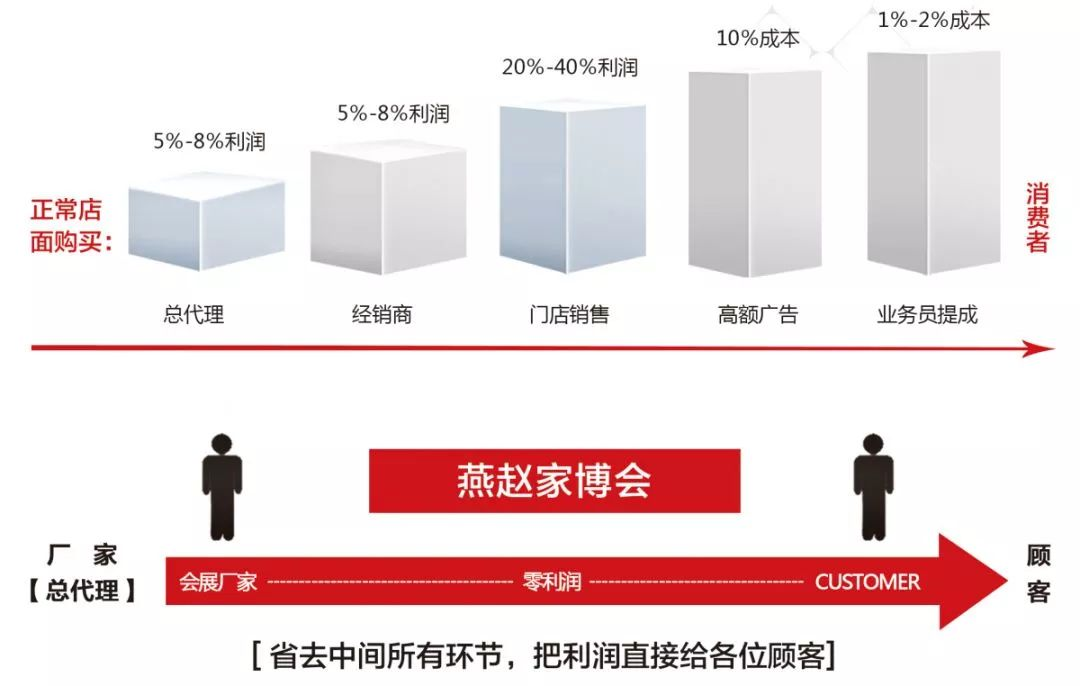多重抽奖乐翻天，贴心服务暖人心

除了众商家隆重推出各种特惠活动外，家博会组委会也重磅推出了“订单抽红包”、“整点抽大奖”、“微信摇一摇”等精彩缤纷的抽奖活动，凡到场的业主均可参与“微信摇一摇”，现场下订单即可参与“订单抽红包”，还可参与“整点抽大奖”，赢取家用电器、家纺家饰等超值奖品，让您奖品拿到手软。同时，为了各位业主快捷方便到达展会现场，燕赵家博会组委会在怀特商城、博物馆、天山海世界、新百广场、益友百货五大商圈设置了免费乘车点，凭家博会门票即可免费乘坐至展会现场；逛展选购可是个力气活，组委会为提前预约VIP门票的业主准备了精美午餐，让每位逛展的业主元气满满的选购装修材料！

装新家不用愁，农业银行助力家博

燕赵家博会携手中国农业银行打造“首届农行家装节”，推出“农行家装分期”惠民服务，让家装不再等待！不仅解绝“装修费用”的燃眉之急，装修公司、家电、 家具 、装饰建材更以最低价一站购齐，让家装不局促，安心打造梦想小屋！火速来燕赵家博会，幸福早到来！好品牌高品质，超强售后保证

燕赵家博会秉承客户至上原则，对参展产品和品牌进行严格筛选，所有在家博会上展出的产品，都是经过市场长久考验，并具有良好社会口碑；凡参展的建材家居品牌均是经国家质量部门认证的品牌产品，“三无”及假冒伪劣产品绝不可进场的。所有展出产品商家在举办地区有直接的售后服务点，能第一时间解决售后服务问题；另外，将享受燕赵家博会组委会全程售后协助保障，市民可以放心地到家博会现场选购自己中意的家装产品。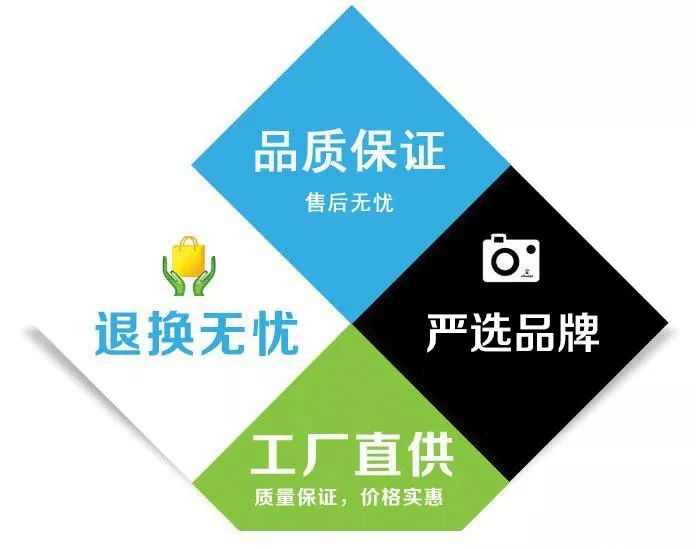2019第十三届燕赵家博会，广大装修业主在品牌、价格和售后服务等方面都将得到真正的保护，尽可放心采购，享受到“省心、省力、省时、省钱”的购物体验。作为开春的第一场家装盛宴，燕赵家博会必将掀起家居装修市场的采购高潮。

第十三届燕赵家博会

展会时间：2019年2月22日-24日

展会地点：石家庄国际会展中心（正定）

官方微信：燕赵家博会

官方微博：@河北燕赵家博会

`声明：本文由入驻焦点开放平台的作者撰写，除焦点官方账号外，观点仅代表作者本人，不代表焦点立场错误信息举报电话： 400-099-0099，邮箱：jubao@vip.sohu.com，或点此进行意见反馈，或点此进行举报投诉。`A B C D E F G H J K L M N P Q R S T W X Y Z
A - B - C - D - E
• A
• 鞍山
• 安庆
• 安阳
• 安顺
• 安康
• 澳门
• B
• 北京
• 保定
• 包头
• 巴彦淖尔
• 本溪
• 蚌埠
• 亳州
• 滨州
• 北海
• 百色
• 巴中
• 毕节
• 保山
• 宝鸡
• 白银
• 巴州
• C
• 承德
• 沧州
• 长治
• 赤峰
• 朝阳
• 长春
• 常州
• 滁州
• 池州
• 长沙
• 常德
• 郴州
• 潮州
• 崇左
• 重庆
• 成都
• 楚雄
• 昌都
• 慈溪
• 常熟
• D
• 大同
• 大连
• 丹东
• 大庆
• 东营
• 德州
• 东莞
• 德阳
• 达州
• 大理
• 德宏
• 定西
• 儋州
• 东平
• E
• 鄂尔多斯
• 鄂州
• 恩施
F - G - H - I - J
• F
• 抚顺
• 阜新
• 阜阳
• 福州
• 抚州
• 佛山
• 防城港
• G
• 赣州
• 广州
• 桂林
• 贵港
• 广元
• 广安
• 贵阳
• 固原
• H
• 邯郸
• 衡水
• 呼和浩特
• 呼伦贝尔
• 葫芦岛
• 哈尔滨
• 黑河
• 淮安
• 杭州
• 湖州
• 合肥
• 淮南
• 淮北
• 黄山
• 菏泽
• 鹤壁
• 黄石
• 黄冈
• 衡阳
• 怀化
• 惠州
• 河源
• 贺州
• 河池
• 海口
• 红河
• 汉中
• 海东
• 怀来
• I
• J
• 晋中
• 锦州
• 吉林
• 鸡西
• 佳木斯
• 嘉兴
• 金华
• 景德镇
• 九江
• 吉安
• 济南
• 济宁
• 焦作
• 荆门
• 荆州
• 江门
• 揭阳
• 金昌
• 酒泉
• 嘉峪关
K - L - M - N - P
• K
• 开封
• 昆明
• 昆山
• L
• 廊坊
• 临汾
• 辽阳
• 连云港
• 丽水
• 六安
• 龙岩
• 莱芜
• 临沂
• 聊城
• 洛阳
• 漯河
• 娄底
• 柳州
• 来宾
• 泸州
• 乐山
• 六盘水
• 丽江
• 临沧
• 拉萨
• 林芝
• 兰州
• 陇南
• M
• 牡丹江
• 马鞍山
• 茂名
• 梅州
• 绵阳
• 眉山
• N
• 南京
• 南通
• 宁波
• 南平
• 宁德
• 南昌
• 南阳
• 南宁
• 内江
• 南充
• P
• 盘锦
• 莆田
• 平顶山
• 濮阳
• 攀枝花
• 普洱
• 平凉
Q - R - S - T - W
• Q
• 秦皇岛
• 齐齐哈尔
• 衢州
• 泉州
• 青岛
• 清远
• 钦州
• 黔南
• 曲靖
• 庆阳
• R
• 日照
• 日喀则
• S
• 石家庄
• 沈阳
• 双鸭山
• 绥化
• 上海
• 苏州
• 宿迁
• 绍兴
• 宿州
• 三明
• 上饶
• 三门峡
• 商丘
• 十堰
• 随州
• 邵阳
• 韶关
• 深圳
• 汕头
• 汕尾
• 三亚
• 三沙
• 遂宁
• 山南
• 商洛
• 石嘴山
• T
• 天津
• 唐山
• 太原
• 通辽
• 铁岭
• 泰州
• 台州
• 铜陵
• 泰安
• 铜仁
• 铜川
• 天水
• 天门
• W
• 乌海
• 乌兰察布
• 无锡
• 温州
• 芜湖
• 潍坊
• 威海
• 武汉
• 梧州
• 渭南
• 武威
• 吴忠
• 乌鲁木齐
X - Y - Z
• X
• 邢台
• 徐州
• 宣城
• 厦门
• 新乡
• 许昌
• 信阳
• 襄阳
• 孝感
• 咸宁
• 湘潭
• 湘西
• 西双版纳
• 西安
• 咸阳
• 西宁
• 仙桃
• 西昌
• Y
• 运城
• 营口
• 盐城
• 扬州
• 鹰潭
• 宜春
• 烟台
• 宜昌
• 岳阳
• 益阳
• 永州
• 阳江
• 云浮
• 玉林
• 宜宾
• 雅安
• 玉溪
• 延安
• 榆林
• 银川
• Z
• 张家口
• 镇江
• 舟山
• 漳州
• 淄博
• 枣庄
• 郑州
• 周口
• 驻马店
• 株洲
• 张家界
• 珠海
• 湛江
• 肇庆
• 中山
• 自贡
• 资阳
• 遵义
• 昭通
• 张掖
• 中卫

1室1厅1厨1卫1阳台

1
2
3
4
5

0
1
2

1

1

0
1
2
3报名成功，资料已提交审核A B C D E F G H J K L M N P Q R S T W X Y Z
A - B - C - D - E
• A
• 鞍山
• 安庆
• 安阳
• 安顺
• 安康
• 澳门
• B
• 北京
• 保定
• 包头
• 巴彦淖尔
• 本溪
• 蚌埠
• 亳州
• 滨州
• 北海
• 百色
• 巴中
• 毕节
• 保山
• 宝鸡
• 白银
• 巴州
• C
• 承德
• 沧州
• 长治
• 赤峰
• 朝阳
• 长春
• 常州
• 滁州
• 池州
• 长沙
• 常德
• 郴州
• 潮州
• 崇左
• 重庆
• 成都
• 楚雄
• 昌都
• 慈溪
• 常熟
• D
• 大同
• 大连
• 丹东
• 大庆
• 东营
• 德州
• 东莞
• 德阳
• 达州
• 大理
• 德宏
• 定西
• 儋州
• 东平
• E
• 鄂尔多斯
• 鄂州
• 恩施
F - G - H - I - J
• F
• 抚顺
• 阜新
• 阜阳
• 福州
• 抚州
• 佛山
• 防城港
• G
• 赣州
• 广州
• 桂林
• 贵港
• 广元
• 广安
• 贵阳
• 固原
• H
• 邯郸
• 衡水
• 呼和浩特
• 呼伦贝尔
• 葫芦岛
• 哈尔滨
• 黑河
• 淮安
• 杭州
• 湖州
• 合肥
• 淮南
• 淮北
• 黄山
• 菏泽
• 鹤壁
• 黄石
• 黄冈
• 衡阳
• 怀化
• 惠州
• 河源
• 贺州
• 河池
• 海口
• 红河
• 汉中
• 海东
• 怀来
• I
• J
• 晋中
• 锦州
• 吉林
• 鸡西
• 佳木斯
• 嘉兴
• 金华
• 景德镇
• 九江
• 吉安
• 济南
• 济宁
• 焦作
• 荆门
• 荆州
• 江门
• 揭阳
• 金昌
• 酒泉
• 嘉峪关
K - L - M - N - P
• K
• 开封
• 昆明
• 昆山
• L
• 廊坊
• 临汾
• 辽阳
• 连云港
• 丽水
• 六安
• 龙岩
• 莱芜
• 临沂
• 聊城
• 洛阳
• 漯河
• 娄底
• 柳州
• 来宾
• 泸州
• 乐山
• 六盘水
• 丽江
• 临沧
• 拉萨
• 林芝
• 兰州
• 陇南
• M
• 牡丹江
• 马鞍山
• 茂名
• 梅州
• 绵阳
• 眉山
• N
• 南京
• 南通
• 宁波
• 南平
• 宁德
• 南昌
• 南阳
• 南宁
• 内江
• 南充
• P
• 盘锦
• 莆田
• 平顶山
• 濮阳
• 攀枝花
• 普洱
• 平凉
Q - R - S - T - W
• Q
• 秦皇岛
• 齐齐哈尔
• 衢州
• 泉州
• 青岛
• 清远
• 钦州
• 黔南
• 曲靖
• 庆阳
• R
• 日照
• 日喀则
• S
• 石家庄
• 沈阳
• 双鸭山
• 绥化
• 上海
• 苏州
• 宿迁
• 绍兴
• 宿州
• 三明
• 上饶
• 三门峡
• 商丘
• 十堰
• 随州
• 邵阳
• 韶关
• 深圳
• 汕头
• 汕尾
• 三亚
• 三沙
• 遂宁
• 山南
• 商洛
• 石嘴山
• T
• 天津
• 唐山
• 太原
• 通辽
• 铁岭
• 泰州
• 台州
• 铜陵
• 泰安
• 铜仁
• 铜川
• 天水
• 天门
• W
• 乌海
• 乌兰察布
• 无锡
• 温州
• 芜湖
• 潍坊
• 威海
• 武汉
• 梧州
• 渭南
• 武威
• 吴忠
• 乌鲁木齐
X - Y - Z
• X
• 邢台
• 徐州
• 宣城
• 厦门
• 新乡
• 许昌
• 信阳
• 襄阳
• 孝感
• 咸宁
• 湘潭
• 湘西
• 西双版纳
• 西安
• 咸阳
• 西宁
• 仙桃
• 西昌
• Y
• 运城
• 营口
• 盐城
• 扬州
• 鹰潭
• 宜春
• 烟台
• 宜昌
• 岳阳
• 益阳
• 永州
• 阳江
• 云浮
• 玉林
• 宜宾
• 雅安
• 玉溪
• 延安
• 榆林
• 银川
• Z
• 张家口
• 镇江
• 舟山
• 漳州
• 淄博
• 枣庄
• 郑州
• 周口
• 驻马店
• 株洲
• 张家界
• 珠海
• 湛江
• 肇庆
• 中山
• 自贡
• 资阳
• 遵义
• 昭通
• 张掖
• 中卫• 手机• 分享
• 设计
免费设计
• 计算器
装修计算器
• 入驻
合作入驻
• 联系
联系我们
• 置顶
返回顶部